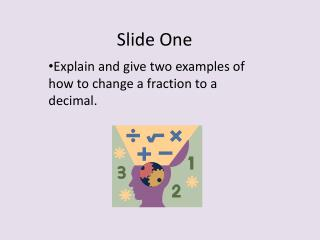Download PresentationSlide One

Slide One - PowerPoint PPT Presentation

Slide One. Explain and give two examples of how to change a fraction to a decimal. Slide Two:. Explain and give two examples of how to change a decimal to a percent. Slide Three:. Explain and give two examples of how to change a percent to a decimal. Slide Four:.I am the owner, or an agent authorized to act on behalf of the owner, of the copyrighted work described.
Download PresentationSlide One

Download Policy: Content on the Website is provided to you AS IS for your information and personal use and may not be sold / licensed / shared on other websites without getting consent from its author.While downloading, if for some reason you are not able to download a presentation, the publisher may have deleted the file from their server.

- - - - - - - - - - - - - - - - - - - - - - - - - - E N D - - - - - - - - - - - - - - - - - - - - - - - - - -
Presentation Transcript
1. Slide One Explain and give two examples of how to change a fraction to a decimal.

2. Slide Two: • Explain and give two examples of how to change a decimal to a percent.

3. Slide Three: • Explain and give two examples of how to change a percent to a decimal.

4. Slide Four: Explain and five two examples of how to change a decimal to a fraction.

5. Slide Five Solve these scientific notation problems. Answer each questions by converting the given standard form to the correct scientific notation. 378000 0.00934 0.000000983 1.9284 34.903 0.000078

6. Slide SixAnswer each questions by converting the given scientific notation to thecorrect standard form. 2.345 x 10 4.56343 x 10 7.904 x 10 3.5093 x 10 2.309 x 10

7. Slide Seven

8. Slide Eight • Explain how to add integers and solve the equations below. • 16 + (-2) • -23 + (-18) • -8 + 3

9. Slide Nine • Explain how to subtract integers and solve the examples below. • -7 – (-8) • 9 – (-3) • 15-30

10. Slide Ten • Explain how to multiply and divide integers and solve the problems below. • -5(4) • -8 (-1/2) • 30.4/-2 • -65/-5

11. Slide ElevenSolve the exponent problems below.

12. Slide Twelve Negative Exponents: Complete

13. Slide Thirteen • Explain order of operations (PEMDAS) and solve the problems below.

14. Slide Fourteen • Explain how to put these numbers in order from least to greatest and give the answer. • Hint!! Convert them all to decimals and whole numbers.